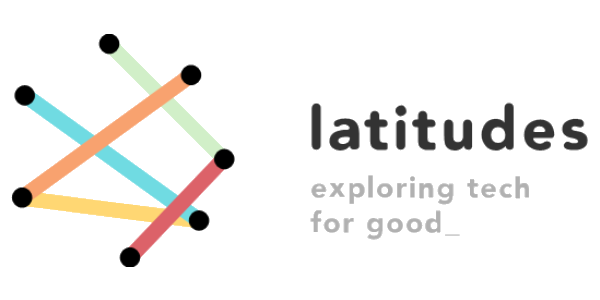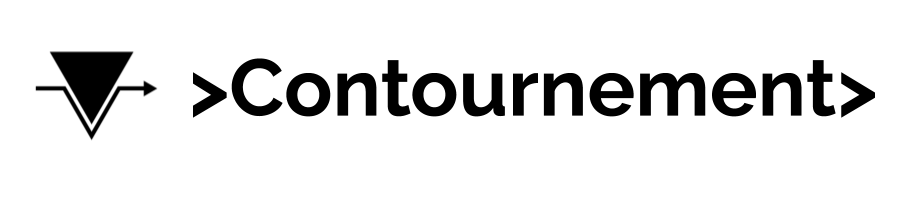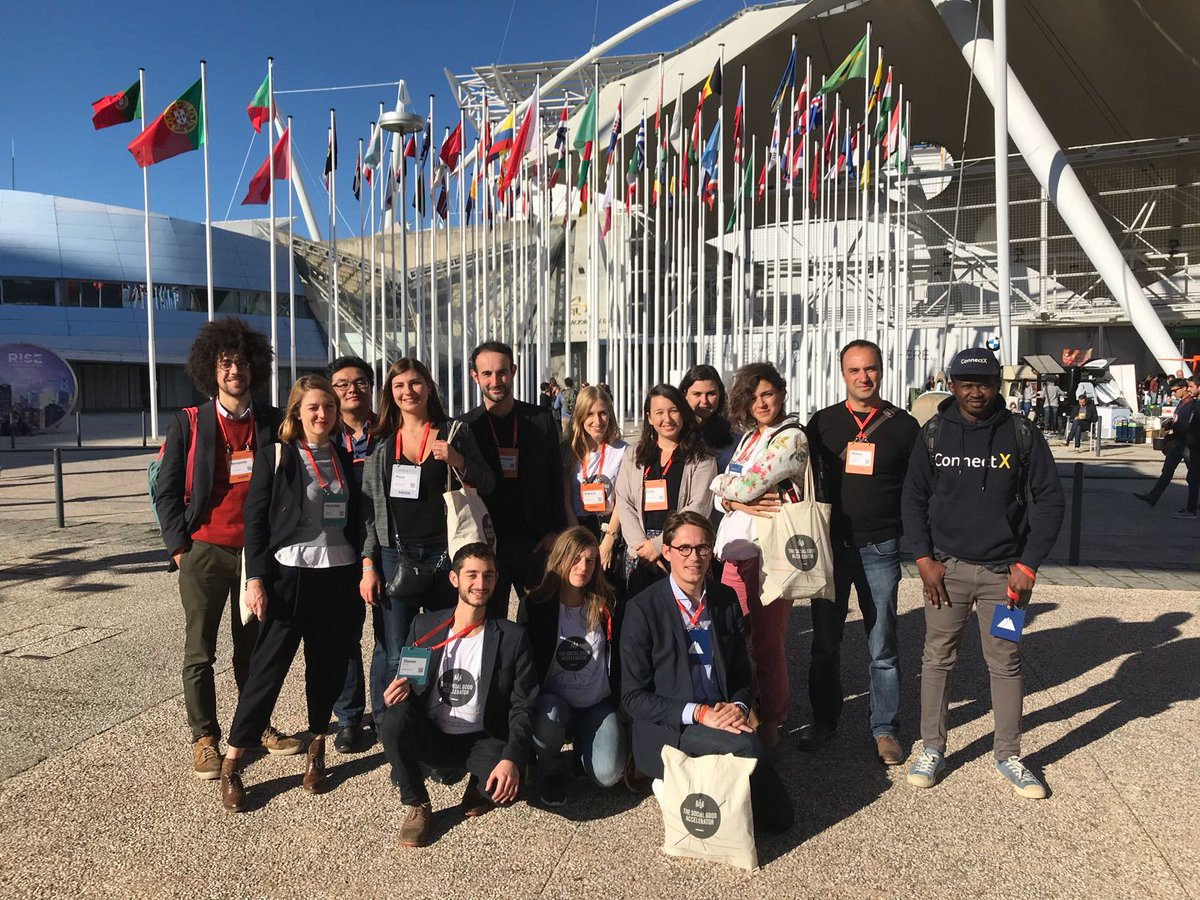Sélectionner une pageThe Social Good Accelerator Community Academy is a cycle of webinars and resource tips to promote knowledge, skills-building and benchmark of good practices. Peer to peer contents suggested by our active members to raise awareness, and share knowledge with our community on Digital culture and tools, with a « Maker » « DIY » spirit.

## DISCOVER OUR MEMBERS CONTENTS…` `# SOCIAL INNOVATION VILLAGE @ WEB SUMMIT 2018

#### The “Social Innovation Village” is a collective non-profit initiative, launched by the Social Good Accelerator, supported by European Commission Directorate General for Research and Innovation and private partners such as Fondation La France s’engage, Accenture, Fondation Groupe EDF and Société Générale Directorate of Innovation. The Village is the place where social meets tech, and tech meets social. We aim to diffuse knowledge about and accelerate social innovation in the EU, the Social Innovation Village hosts 24 social innovators and representative stakeholders.

Our Web Summit 2018 conference

``` ```

``` ```

``` ```

``` ```

``` ```

``` ```

``` ```

``` ```

``` ```

``` ```

``` ```

``` ```

``` ```

``` ```

``` ```

``` ```

``` ```

``` ```

``` ```

``` ```

``` ```

``` ```

``` ```

``` ```

``` ```

``` ```

``` ```

``` ```

``` ```

``` ```

``` ```

``` ```

``` ```

``` ```

``` ```

``` ```

``` ```

``` ```

``` ```

``` ```

``` ```

``` ```

``` ```

``` ```

``` ```

``` ```

``` ```

``` ```

``` ```

``` ```

``` ```

``` ```

``` ```

``` ```

``` ```

``` ```

``` ```

``` ```

``` ```

``` ```

``` ```

``` ```

``` ```

``` ```

``` ```

``` ```

``` ```

``` ```

``` ```

``` ```

``` ```

« More Social Good In Tech, More Tech in Social Good »

``` ```

``` ```

``` ```

``` ```

``` ```

``` ```

``` ```

``` ```

``` ```

``` ```

``` ```

``` ```

``` ```

``` ```

``` ```

``` ```

``` ```

``` ```

``` ```

``` ```

``` ```

``` ```

``` ```

``` ```

``` ```

``` ```

``` ```

``` ```

``` ```

``` ```

``` ```

``` ```

``` ```

``` ```

``` ```

``` ```

``` ```

``` ```

``` ```

``` ```

``` ```

``` ```

``` ```

``` ```

``` ```

``` ```

``` ```

With Carlos Moedas, European Commisonner for Research, Science and Innovation; Mounir Mahjoubi, French Secretary of State for Digital Affairs ; Monique Morrow, President of The Humanized Internet ; Jeanne Bretécher, President, The Social Good Accelerator

``` ```

``` ```

``` ```

``` ```

``` ```

``` ```

``` ```

``` ```

``` ```

``` ```

``` ```

``` ```

``` ```

``` ```

``` ```

``` ```

``` ```

``` ```

``` ```

``` ```

``` ```

``` ```

``` ```

``` ```

``` ```

``` ```

``` ```

``` ```

``` ```

``` ```

``` ```

``` ```

``` ```

``` ```

``` ```

``` ```

``` ```

``` ```

``` ```

``` ```

``` ```

``` ```

``` ```

``` ```

``` ```

``` ```

``` ```

Social Innovation Village Projects at Web Summit 2018

Our 24 projects could not have been exhibited whithout the kind support of :

_____________________________________________________________________________________________________________________________________

``` ```

``` ```

``` ```

``` ```

``` ```

``` ```

``` ```

``` ```

``` ```

``` ```

``` ```

``` ```

``` ```

``` ```

``` ```

``` ```

``` ```

``` ```

``` ```

``` ```

``` ```

``` ```

``` ```

``` ```

``` ```

``` ```

``` ```

``` ```

``` ```

``` ```

``` ```

``` ```

``` ```

``` ```

``` ```

``` ```

``` ```

``` ```

``` ```

``` ```

``` ```

``` ```

``` ```

``` ```

``` ```

``` ```

``` ```

``` ```

# Delegation 2017 at Web Summit 2017

``` ```

``` ```

``` ```

``` ```

``` ```

``` ```

``` ```

``` ```

``` ```

``` ```

``` ```

``` ```

``` ```

``` ```

``` ```

``` ```

``` ```

``` ```

``` ```

``` ```

``` ```

``` ```

``` ```

``` ```

``` ```

``` ```

``` ```

``` ```

``` ```

``` ```

``` ```

``` ```

``` ```

``` ```

``` ```

``` ```

``` ```

``` ```

``` ```

``` ```

``` ```

``` ```

``` ```

``` ```

``` ```

``` ```

``` ```

``` ```

``` ```

``` ```

``` ```

``` ```

``` ```

``` ```

``` ```

``` ```

``` ```

``` ```

``` ```

``` ```

``` ```

``` ```

``` ```

``` ```

``` ```

``` ```

``` ```

``` ```

``` ```

``` ```

``` ```

``` ```

``` ```

``` ```

``` ```

``` ```

``` ```

``` ```

``` ```

``` ```

``` ```

``` ```

``` ```

``` ```

``` ```

``` ```

``` ```

``` ```

``` ```

``` ```

``` ```

``` ```

``` ```

``` ```

``` `````` ```

``` ```

``` ```

``` ```

``` ```

``` ```

``` ```

``` ```

``` ```

``` ```

``` ```

``` ```

``` ```

``` ```

``` ```

``` ```

``` ```

``` ```

``` ```

``` ```

``` ```

``` ```

``` ```

``` ```

``` ```

``` ```

``` ```

``` ```

``` ```

``` ```

``` ```

``` ```

``` ```

``` ```

``` ```

``` ```

``` ```

``` ```

``` ```

``` ```

``` ```

``` ```

``` ```

``` ```

``` ```

``` ```

``` ```

``` ```

``` ```

``` ```

``` ```

``` ```

``` ```

``` ```

``` ```

``` ```

``` ```

``` ```

``` ```

``` ```

``` ```

``` ```

``` ```

``` ```

``` ```

``` ```

``` ```

``` ```

``` ```

``` ```

``` ```

``` ```

``` ```

``` ```

``` ```

``` ```

``` ```

``` ```

``` ```

``` ```

``` ```

``` ```

``` ```

``` ```

``` ```

``` ```

``` ```

``` ```

``` ```

``` ```

``` ```

``` ```

``` ```

``` ```

``` ```

``` ```

``` ```

``` ```

``` ```

``` ```

``` ```

``` ```

``` ```

``` ```

``` ```

``` ```

``` ```

``` ```

``` ```

``` ```

``` ```

``` ```

``` ```

``` ```

``` ```

``` ```

``` ```

``` ```

``` ```

``` ```

``` ```

``` ```

``` ```

``` ```

``` ```

``` ```

``` ```

``` ```

``` ```

``` ```

``` ```

``` ```

``` ```

``` ```

``` ```

``` ```

``` ```

``` ```

``` ```

``` ```

``` ```

``` ```

``` ```

``` ```

``` ```

``` ```

``` ```

``` ```

``` ```

``` ```

``` ```

``` ```

``` ```

``` ```

``` ```

``` ```

``` ```

``` ```

``` ```

``` ```

``` ```

``` ```

``` ```

``` ```

``` ```

``` ```

``` ```

``` ```

``` ```

``` ```

``` ```

``` ```

``` ```

``` ```

``` ```

``` ```

``` ```

``` ```

``` ```

``` ```

``` ```

``` ```

``` ```

``` ```

``` ```

``` ```

``` ```

``` ```

``` ```

``` ```

``` ```

``` ```

``` ```

``` ```

``` ```

``` ```

``` ```

``` ```

``` ```

``` ```

``` ```

``` ```

``` ```

``` ```

``` ```

``` ```

``` ```

``` ```

``` ```

``` ```

``` ```

``` ```

``` ```

``` ```

``` ```

``` ```

``` ```

``` ```

``` ```

``` ```

``` ```

``` ```

``` ```

``` ```

``` ```

``` ```

``` ```

``` ```

``` ```

``` ```

``` ```

``` ```

``` ```

``` ```

``` ```

``` ```

``` ```

``` ```

``` ```

``` ```

``` ```

``` ```

``` ```

``` ```

``` ```

``` ```

``` ```

``` ```

``` ```

``` ```

``` ```

``` ```

``` ```

``` ```

``` ```

``` ```

``` ```

``` ```

``` ```

``` ```

``` ```

``` ```

``` ```

``` ```

``` ```

``` ```

``` ```

``` ```

``` ```

``` ```

``` ```

``` ```

``` ```

``` ```

``` ```

``` ```

``` ```

``` ```

``` ```

``` ```

``` ```

``` ```

``` ```

``` ```

``` ```

``` ```

``` ```

``` ```

``` ```

``` ```

``` ```

``` ```

``` ```

``` ```

``` ```

``` ```

``` ```

``` ```

``` ```

``` ```

``` ```

``` ```

``` ```

``` ```

``` ```

``` ```

``` ```

``` ```

``` ```

``` ```

``` ```

``` ```

``` ```

``` ```

``` ```

``` ```

``` ```

``` ```

``` ```

``` ```

``` ```

``` ```

``` ```

``` ```

``` ```

``` ```

``` ```

``` ```

``` ```

``` ```

``` ```

``` ```

``` ```

``` ```

``` ```

``` ```

``` ```

``` ```

``` ```

``` ```

``` ```

``` ```

``` ```

``` ```

``` ```

``` ```

``` ```

``` ```

``` ```

``` ```

``` ```

``` ```

``` ```

``` ```

``` ```

``` ```

``` ```

``` ```

``` ```

``` ```

``` ```

``` ```

``` ```

``` ```

``` ```

``` ```

``` ```

``` ```

``` ```

``` ```

``` ```

``` ```

``` ```

``` ```

``` ```

``` ```

``` ```

``` ```

``` ```

``` ```

``` ```

``` ```

``` ```

``` ```

``` ```

``` ```

``` ```

``` ```

``` ```

``` ```

``` ```

``` ```

``` ```

``` ```

``` ```

``` ```

``` ```

``` ```

``` ```

``` ```

``` ```

``` ```

``` ```

``` ```

``` ```

``` ```

``` ```

``` ```

``` ```

``` ```

``` ```

``` ```

``` ```

``` ```

``` ```

``` ```

``` ```

``` ```

``` ```

``` ```

``` ```

``` ```

``` ```

``` ```

``` ```

``` ```

``` ```

``` ```

``` ```

``` ```

``` ```

``` ```

``` ```

``` ```

``` ```

``` ```

``` ```

``` ```

``` ```

``` ```

``` ```

``` ```

``` ```

``` ```

``` ```

``` ```

``` ```

``` ```

``` ```

``` ```

``` ```

``` ```

``` ```

``` ```

``` ```

``` ```

``` ```

``` ```

``` ```

``` ```

``` ```

``` ```

``` ```

``` ```

``` ```

``` ```

``` ```

``` ```

``` ```

``` ```

``` ```

``` ```

``` ```

``` ```

``` ```

``` ```

``` ```

``` ```

``` ```

``` ```

``` ```

``` ```

``` ```

``` ```

``` ```

``` ```

``` ```

``` ```

``` ```

``` ```

``` ```

``` ```

``` ```

``` ```

``` ```

``` ```

``` ```

``` ```

``` ```

``` ```

``` ```

``` ```

``` ```

``` ```

``` ```

``` ```

``` ```

``` ```

``` ```

``` ```

``` ```

``` ```

``` ```

``` ```

``` ```

``` ```

``` ```

``` ```

``` ```

``` ```

``` ```

``` ```

``` ```

``` ```

``` ```

``` ```

``` ```

``` ```

``` ```

``` ```

``` ```

``` ```

``` ```

``` ```

``` ```

``` ```

``` ```

``` ```

``` ```

``` ```

``` ```

``` ```

``` ```

``` ```

``` ```

``` ```

``` ```

``` ```

``` ```

``` ```

``` ```

``` ```

``` ```

``` ```

``` ```

``` ```

``` ```

``` ```

``` ```

``` ```

``` ```

``` ```

``` ```

``` ```

``` ```

``` ```

``` ```

``` ```

``` ```

``` ```

``` ```

``` ```

``` ```

``` ```

``` ```

``` ```

``` ```

``` ```

``` ```

``` ```

``` ```

``` ```

``` ```

``` ```

``` ```

``` ```

``` ```

``` ```

``` ```

``` ```

``` ```

``` ```

``` ```

``` ```

``` ```

``` ```

``` ```

``` ```

``` ```

``` ```

``` ```

``` ```

``` ```

``` ```

``` ```

``` ```

``` ```

``` ```

``` ```

``` ```

``` ```

``` ```

``` ```

``` ```

``` ```

``` ```

``` ```

``` ```

``` ```

``` ```

``` ```

``` ```

``` ```

``` ```

``` ```

``` ```

``` ```

``` ```

``` ```

``` ```

``` ```

``` ```

``` ```

``` ```

``` ```

``` ```

``` ```

``` ```

``` ```

``` ```

``` ```

``` ```

``` ```

``` ```

``` ```

``` ```

``` ```

``` ```

``` ```

``` ```

``` ```

``` ```

``` ```

``` ```

``` ```

``` ```

``` ```

``` ```

``` ```

``` ```

``` ```

``` ```

``` ```

``` ```

``` ```

``` ```

``` ```

``` ```

``` ```

``` ```

``` ```

``` ```

``` ```

``` ```

``` ```

``` ```

``` ```

``` ```

``` ```

``` ```

``` ```

``` ```

``` ```

``` ```

``` ```

``` ```

``` ```

``` ```

``` ```

``` ```

``` ```

``` ```

``` ```

``` ```

``` ```

``` ```

``` ```

``` ```

``` ```

``` ```

``` ```

``` ```

``` ```

``` ```

``` ```

``` ```

``` ```

``` ```

``` ```

``` ```

``` ```

``` ```

``` ```

``` ```

``` ```

``` ```

``` ```

``` ```

``` ```

``` ```

``` ```

``` ```

``` ```

``` ```

``` ```

``` ```

``` ```

``` ```

``` ```

``` ```

``` ```

``` ```

``` ```

``` ```

``` ```

``` ```

``` ```

``` ```

``` ```

``` ```

``` ```

``` ```

``` ```

``` ```

``` ```

``` ```

``` ```

``` ```

``` ```

``` ```

``` ```

``` ```

``` ```

``` ```

``` ```

``` ```

``` ```

``` ```

``` ```

``` ```

``` ```